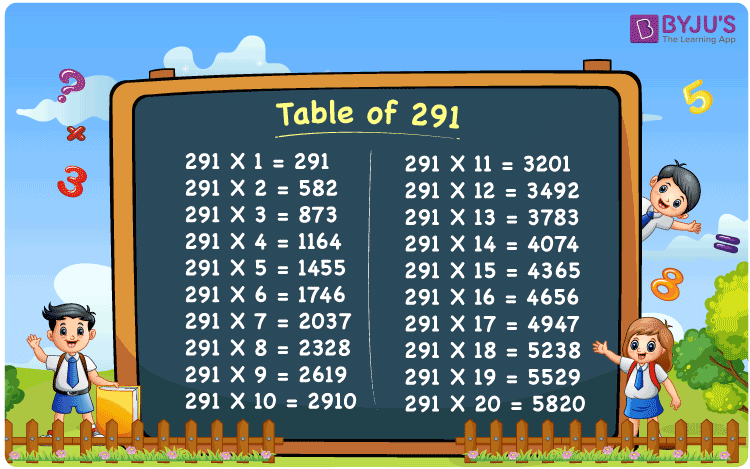Checkout JEE MAINS 2022 Question Paper Analysis : Checkout JEE MAINS 2022 Question Paper Analysis :

# Table of 291

The table of 291 is obtained when we multiply 291 by natural numbers such that 291 is called the multiplication table of 291. However, we know that maths tables can be written using the repeated addition method also. Thus, in this article, you will learn how to write the table of 291 using both multiplication and repeated addition. Also, download the PDF of 291 times table given below:

## Table of 291 Chart## What is the 291 Times Table?

The below table shows the different methods of writing the 291 times table, i.e. using addition and multiplication. Let’s understand how to write the table of 291 up to 10 results using repeated addition and multiplication of 291 by 1, 2, 3, and so on.

 Using multiplication Using repeated addition 291 × 1 = 291 291 291 × 2 = 582 291 + 291 = 582 291 × 3 = 873 291 + 291 + 291 = 873 291 × 4 = 1164 291 + 291 + 291 + 291 = 1164 291 × 5 = 1455 291 + 291 + 291 + 291 + 291 = 1455 291 × 6 = 1746 291 + 291 + 291 + 291 + 291 + 291 = 1746 291 × 7 = 2037 291 + 291 + 291 + 291 + 291 + 291 + 291 = 2037 291 × 8 = 2328 291 + 291 + 291 + 291 + 291 + 291 + 291 + 291 = 2328 291 × 9 = 2619 291 + 291 + 291 + 291 + 291 + 291 + 291 + 291 + 291 = 2619 291 × 10 = 2910 291 + 291 + 291 + 291 + 291 + 291 + 291 + 291 + 291 + 291 = 2910

## Multiplication Table of 291

The multiplication table of 291 is given below up to 20 results and these results are called the first 20 multiples of 291.

 291 × 1 = 291 291 × 2 = 582 291 × 3 = 873 291 × 4 = 1164 291 × 5 = 1455 291 × 6 = 1746 291 × 7 = 2037 291 × 8 = 2328 291 × 9 = 2619 291 × 10 = 2910 291 × 11 = 3201 291 × 12 = 3492 291 × 13 = 3783 291 × 14 = 4074 291 × 15 = 4365 291 × 16 = 4656 291 × 17 = 4947 291 × 18 = 5238 291 × 19 = 5529 291 × 20 = 5820

## Solved Examples

Example 1:

There are 19 boxes of cereal on a shelf. How many boxes are on 291 shelves?

Solution:

Number of shelves = 291

Number of cereal boxes on each shelf = 19

Total number of cereal boxes = 291 × 19 = 5529.

Example 2:

Each crayon costs Rs. 7. How much do 291 crayons cost?

Solution:

Given,

The cost of a crayon = Rs. 7

The cost of 291 such crayons = Rs. 7 × 291 = 2037.

## Frequently Asked Questions on Table of 291

### What is the multiplication table of 291?

The multiplication table of 291 is obtained when 291 is multiplied by numbers 1, 2, 3, and so on. Below are the few entries of the multiplication table of 291:
291 × 1 = 291
291 × 2 = 582
291 × 3 = 873
291 × 4 = 1164
291 × 5 = 1455
291 × 6 = 1746

### In which tables will 291 come?

291 comes as the result in the 3 times table, i.e. 3 × 97 = 291.

### How do you write the value of 291 times 6?

We can write the value of 291 times 6 as given below: 291 × 6 = 1746 Or 291 + 291 + 291 + 291 + 291 + 291 = 1746

### Using the table of 291, find the value of 291 times 9 minus 770.

291 times 9 minus 770 Using the table of 291, 291 times 9 minus 770 = 291 × 9 – 770 = 2619 – 770 = 1849